# Interpret Multiplication Equations as Comparisons

##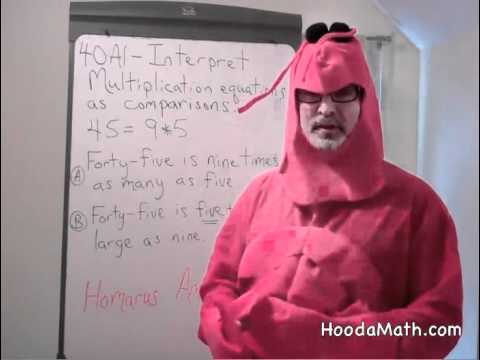By Hoodamath tutorials

Interpret a multiplication equation as a comparison# Finding Unknown Factors in Multiplication Equations: 3.OA.4

##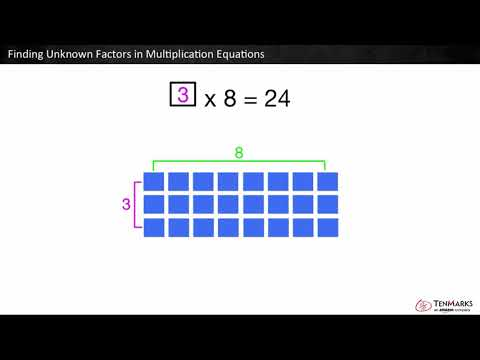By Tenmarks Amazon

Students learn to find unknown factors in multiplication equations using arrays and repeated addition.# One-step equations with multiplication and division

##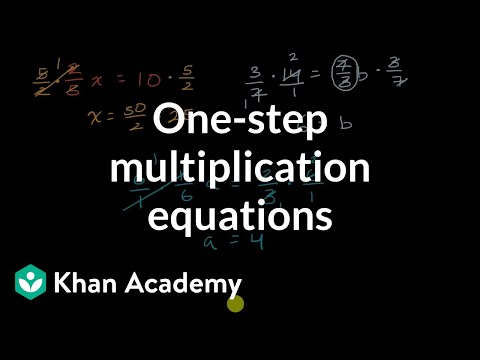By Khan Academy

Remember that what you do to one side, you have to do to the other. Will you multiply or divide both sides to dump the fraction, x/a? Let's do it together.# One-step equations with multiplication and division

##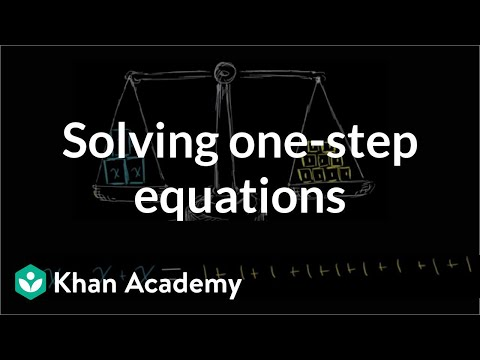By Khan Academy

This equation can be simplified through a single step to solve for the variable. Can you help?# One-step equations with multiplication and division

##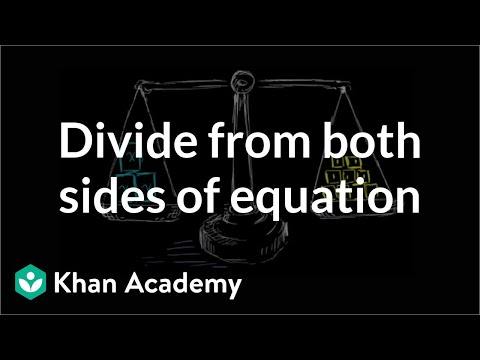By Khan Academy

Let's get a conceptual understanding of why one needs to divide both sides of an equation to solve for a variable.# One-step equations with multiplication and division

##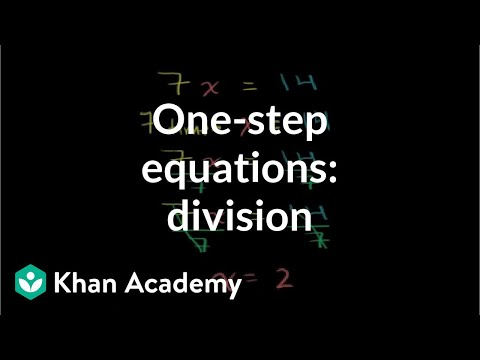By Khan Academy

Let's ease into this, shall we? Here's an introduction to basic algebraic equations of the form ax=b. Remember that you can check to see if you have the right answer by substituting it for the variable!# One-step equations with multiplication and division

##By Khan Academy

This equation can be simplified through a single step to solve for the variable. Can you help?# One-step equations with multiplication and division

##By Khan Academy

Let's ease into this, shall we? Here's an introduction to basic algebraic equations of the form ax=b. Remember that you can check to see if you have the right answer by substituting it for the variable!# One-step equations with multiplication and division

##By Khan Academy

Let's get a conceptual understanding of why one needs to divide both sides of an equation to solve for a variable.# One-step equations with multiplication and division

##By Khan Academy

Remember that what you do to one side, you have to do to the other. Will you multiply or divide both sides to dump the fraction, x/a? Let's do it together.# Systems of equations with elimination (and manipulation) | High School Math | Khan Academy

##By Khan Academy

Khan Academy presents Solving Systems of Equations by Multiplication, an educational video resource on math.# Solve equations with two rational expressions

##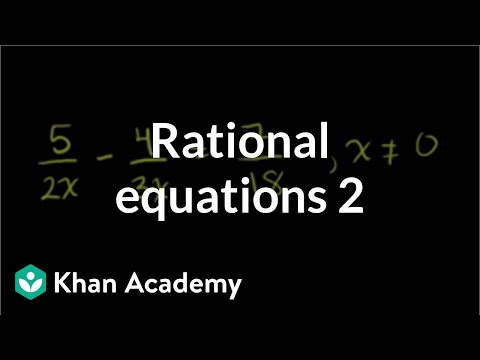By Khan Academy

Sal solves the equation 5/(2x)-4/(3x)=7/18 by first finding the LCM (least common multiple) of 2x and 3x.# Multiplication and Multiplicative Comparison

##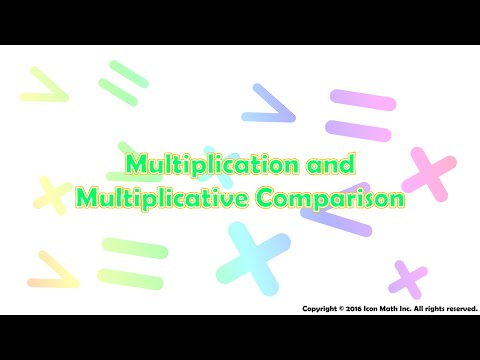By Icon Math

A multiplication equation as a multiplicative comparison.# One-step multiplication and division equations with fractions and decimals

##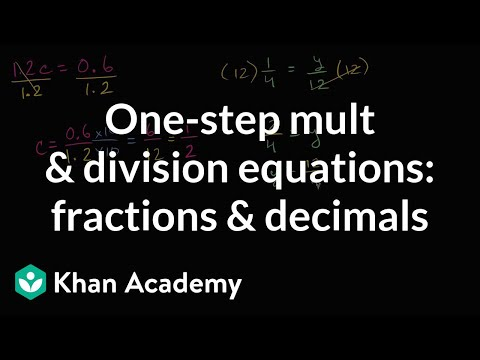By Khan Academy

Learn how to solve equations in one step by multiplying or dividing a number from both sides.ï¿½ï¿½ï¿½ï¿½ï¿½ï¿½ï¿½ï¿½ï¿½ï¿½ï¿½ï¿½These problems involve decimals and fractions.# [4.OA.1-1.0] Multiplication as Comparison - Common Core Standard

##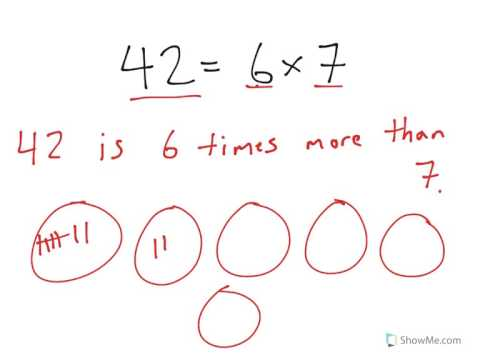By Freckle education

Interpret a multiplication equation as a comparison# Multiplication Comparisons

##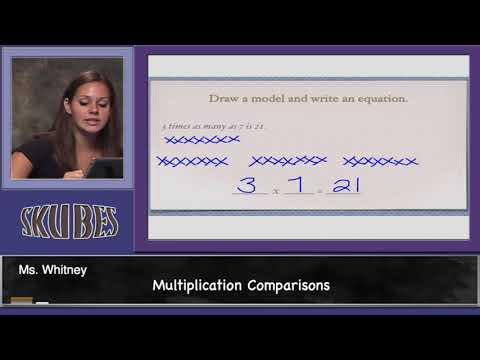By Skubes ed

Interpret a multiplication equation as a comparison# One-step equations with addition and subtraction

##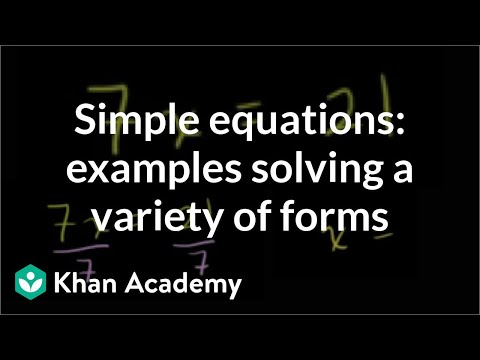By Khan Academy

Some quick examples to practice solving a variety of one step equations. All 4 operations (add, subtract, multiple, divide) are paired with variables.# Unknowns with multiplication and division | Multiplication and division

##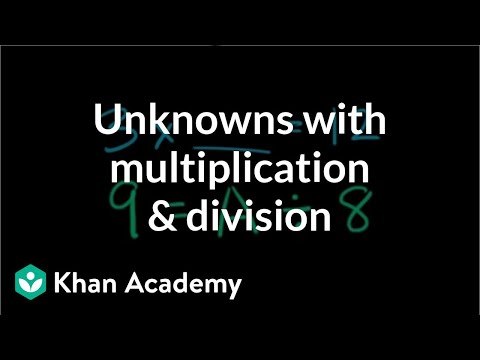By Khan Academy

Find the missing number in multiplication and division equations.# One-step addition and subtraction equations with fractions and decimals

##By Khan Academy

Some quick examples to practice solving a variety of one step equations. All 4 operations (add, subtract, multiple, divide) are paired with variables.# Finding the Missing Factor

##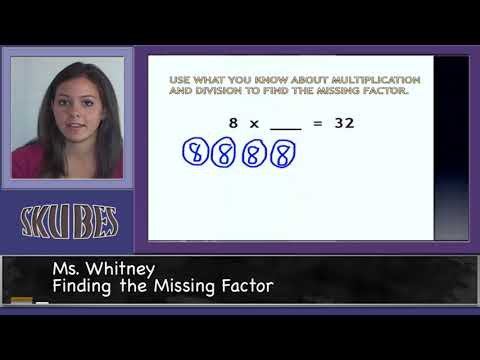By Skubes ed

Determine the unknown whole number in a multiplication or division equation relating three whole numbers. For example, determine the unknown number that makes the equation true in each of the equations 8 x ? = 48, 5 = _ ÷ 3, 6 x 6 = ?